Question

# It has been estimated that 34% of all university students switch majors within their first two...

It has been estimated that 34% of all university students switch majors within their first two years of starting classes. If a random sample of 380 third-year students is taken at a city university, what is an estimate of the probability that 28% or less had switched majors within their first two years? Use Appendix B.1 for the z-values. (Round the z-value to 2 decimal places and the final answer to 4 decimal places.) Probability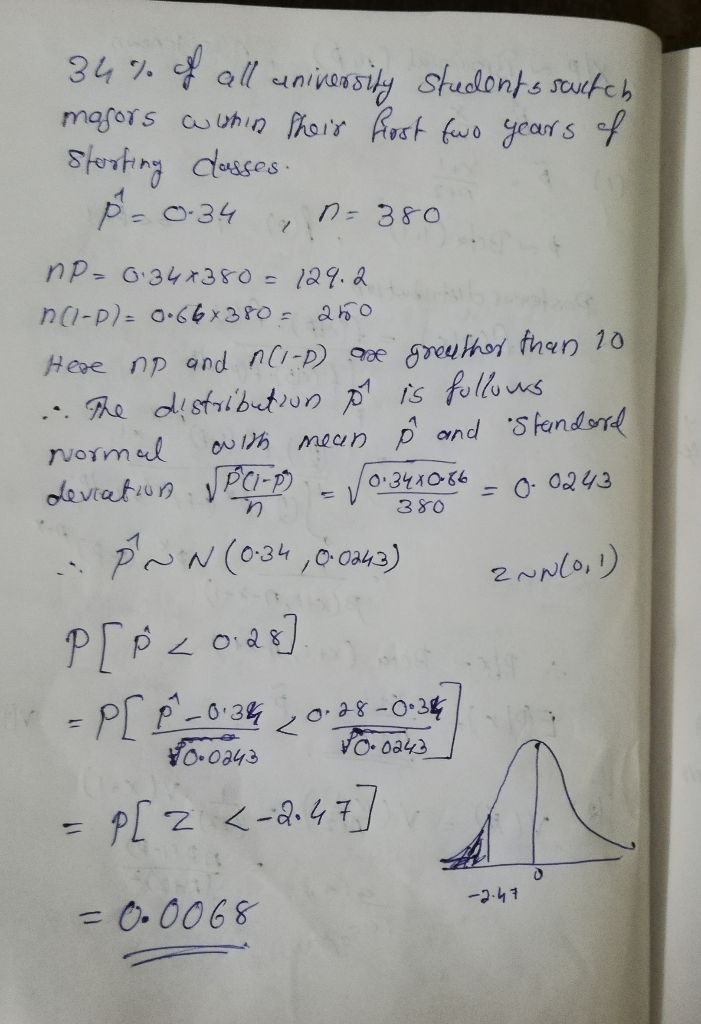#### Earn Coins

Coins can be redeemed for fabulous gifts.

Similar Homework Help Questions
• ### It has been estimated that 27% of all university students switch majors within their first two...

It has been estimated that 27% of all university students switch majors within their first two years of starting classes. If a random sample of 570 third-year students is taken at a city university, what is an estimate of the probability that 23% or less had switched majors within their first two years? Use Appendix B.1 for the z-values. (Round the z-value to 2 decimal places and the final answer to 4 decimal places.) Probability

• ### 13 12 11 10 8 6 7 F 4 5 r3 2 A university class has...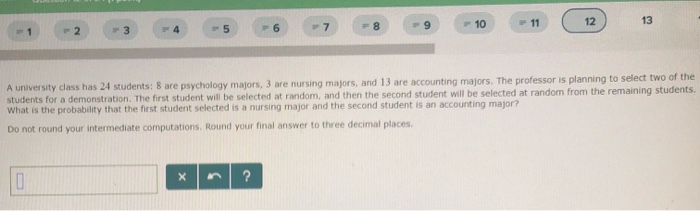13 12 11 10 8 6 7 F 4 5 r3 2 A university class has 24 students: 8 are psychology majors, 3 are nursing majors, and 13 are accounting majors. The professor is planning to select two of the students for a demonstration. The first student will be selected at random, and then the second student will be selected at random from the remaining students. What is the probability that the first student selected is a nursing major and...

• ### Two random samples are taken, one from among first-year students and the other from among fourth-year...

Two random samples are taken, one from among first-year students and the other from among fourth-year students at a public university. Both samples are asked if they favor modifying the student Honor Code. A summary of the sample sizes and number of each group answering yes'' are given below: First-Years (Pop. 1):Fourth-Years (Pop. 2):n1=80,n2=87,x1=35x2=48 Is there evidence, at an α=0.03 level of significance, to conclude that there is a difference in proportions between first-years and fourth-years? Carry out an appropriate...

• ### Two random samples are taken, one from among first-year students and the other from among fourth-year...

Two random samples are taken, one from among first-year students and the other from among fourth-year students at a public university. Both samples are asked if they favor modifying the student Honor Code. A summary of the sample sizes and number of each group answering yes'' are given below: First-Years (Pop. 1): Fourth-Years (Pop. 2): n 1 =84, n 2 =87, x 1 =34 x 2 =37 First-Years (Pop. 1):n1=84,x1=34Fourth-Years (Pop. 2):n2=87,x2=37 Is there evidence, at an α=0.055 α=0.055 level...

• ### David Anderson has been working as a lecturer at Michigan State University for the last three...

David Anderson has been working as a lecturer at Michigan State University for the last three years. He teaches two large sections of introductory accounting every semester. While he uses the same lecture notes in both sections, his students in the first section outperform those in the second section. He believes that students in the first section not only tend to get higher scores, they also tend to have lower variability in scores. David decides to carry out a formal...

• ### (2 points) Two random samples are taken, one from among first year students and the other...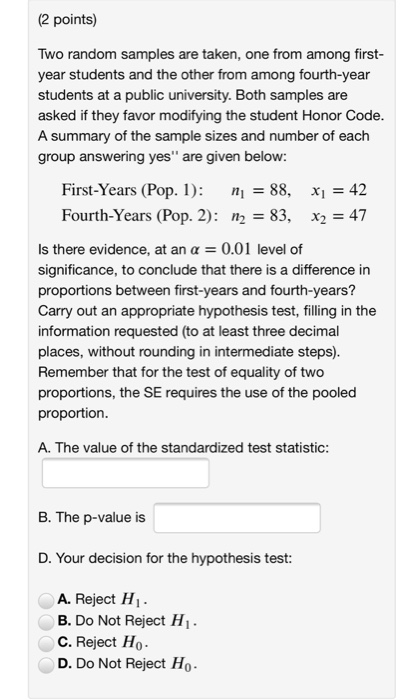(2 points) Two random samples are taken, one from among first year students and the other from among fourth-year students at a public university. Both samples are asked if they favor modifying the student Honor Code A summary of the sample sizes a group answering yes" are given below: nd number of each First-Years (Pop. 1): ni - 88, xi -42 Fourth-Years (Pop. 2): n2 -83, x2 4 Is there evidence, at an a 0.01 level of significance, to conclude...

• ### (2 points) Two random samples are taken, one from among first-year students and the other from...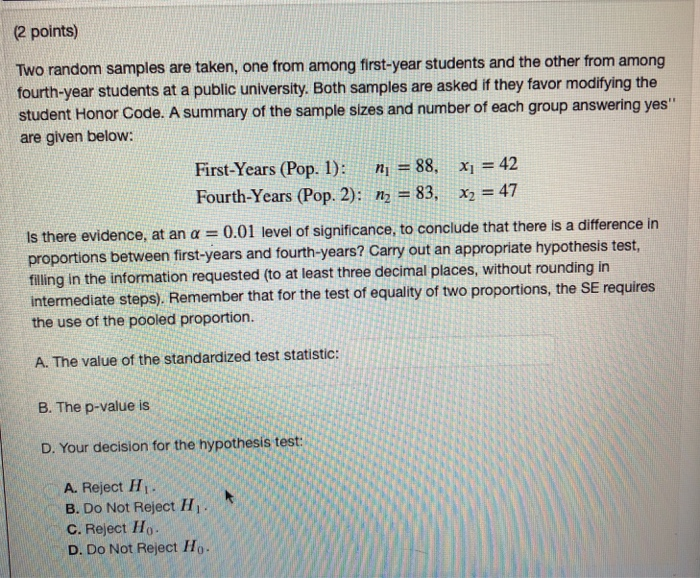(2 points) Two random samples are taken, one from among first-year students and the other from among fourth-year students at a public university. Both samples are asked if they favor modifiying the student Honor Code. A summary of the sample sizes and number of each group answering yes" are given below: First-Years (Pop. 1): n 88. x42 Fourth-Years (Pop. 2): n2-83, x2 = 47 idence, at an α-0.01 level of significance, to conclude that there is a difference in between...

• ### Two random samples are taken, one from among first-year students and the other from among fourth-year...

Two random samples are taken, one from among first-year students and the other from among fourth-year students at a public university. Both samples are asked if they favor modifying the student Honor Code. A summary of the sample sizes and number of each group answering yes'' are given below: First-Years (Pop. 1):Fourth-Years (Pop. 2):?1=88,?2=98,?1=46?2=53 Is there evidence, at an ?=0.04 level of significance, to conclude that there is a difference in proportions between first-years and fourth-years? Carry out an appropriate...

• ### A marketing survey is conducted in which students are to taste two different brands of soft...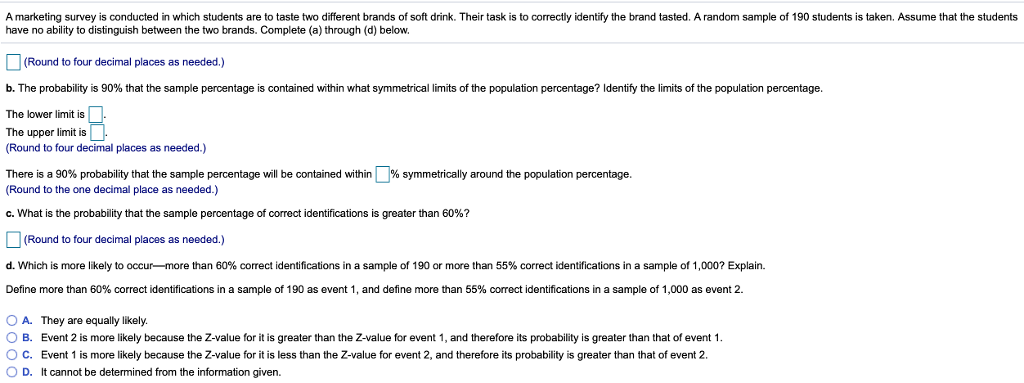A marketing survey is conducted in which students are to taste two different brands of soft drink. Their task is to correctly identify the brand tasted. A random sample of 190 students is taken. Assume that the students have no ability to distinguish between the two brands. Complete (a) through (d) below. (Round to four decimal places as needed.) b The probability is 90% that the sample percentage is contained w thin what symmetrical imrts of he population percentage? dentry...

• ### A public university is considering a change in the way students pay for their undergraduate classes....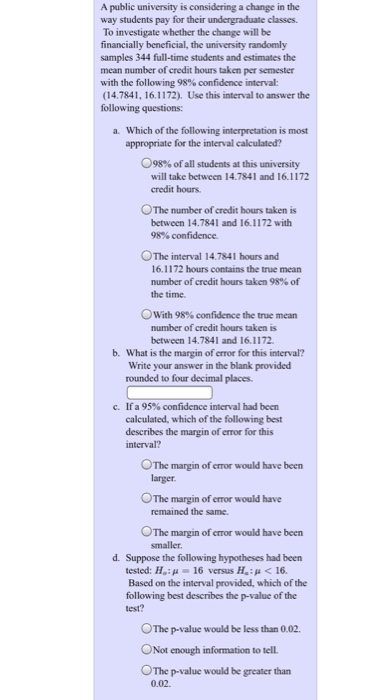A public university is considering a change in the way students pay for their undergraduate classes. To investigate whether the change will be financially beneficial, the university randomly samples 344 full-time students and estimates the mean number of credit hours taken per semester with the following 98% confidence interval: (14.7841, 16.1172), Use this interval to answer the following questions Which of the following interpretation is most appropriate for the interval calculated? a. 098% of all students at this university will...